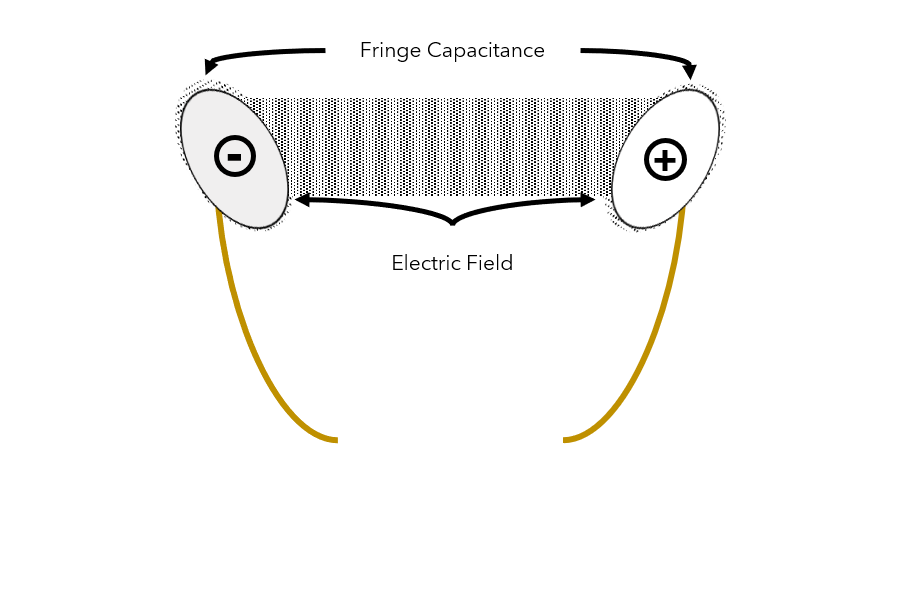Capacitance, along with resistance and inductance, is one of the three fundamental elements of electrical components.

However, in our experience, it is one of the most difficult concepts to understand—especially when trying to relate its impact to energy-driven medical devices.

In this blog post, we’ll try to answer the most common questions we receive about interconnect capacitance.

Recommended: Interconnect Solutions for Medical Devices [The Ultimate Guide]

## What is capacitance?

In simple terms, capacitance measures a component’s (usually a capacitor) ability to store an electrical charge.

To understand the concept of capacitance, it’s useful to know how a component is charged.

Imagine you have two metal plates with a copper wire connected to the back of each plate.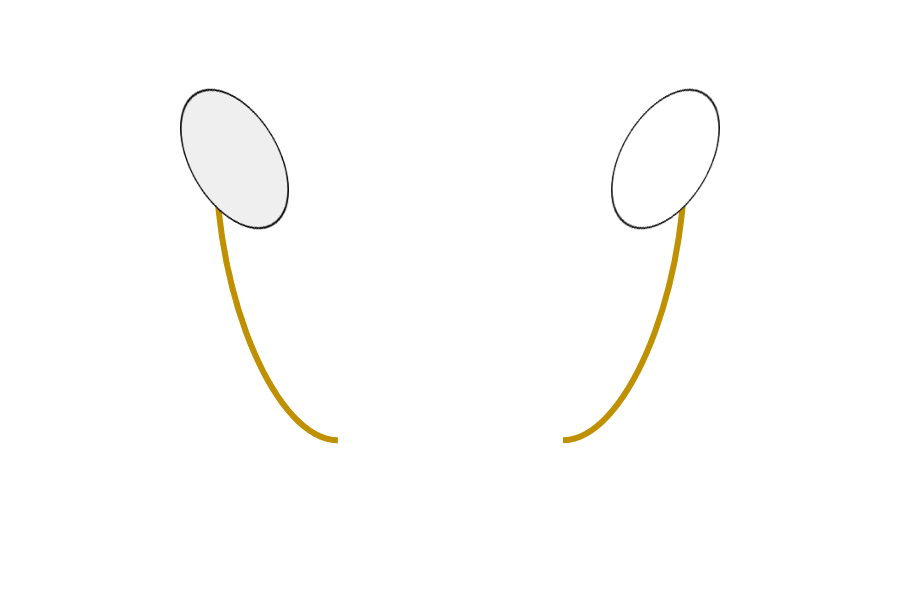Now, imagine you place a standard 1.5 volt battery between the two wires.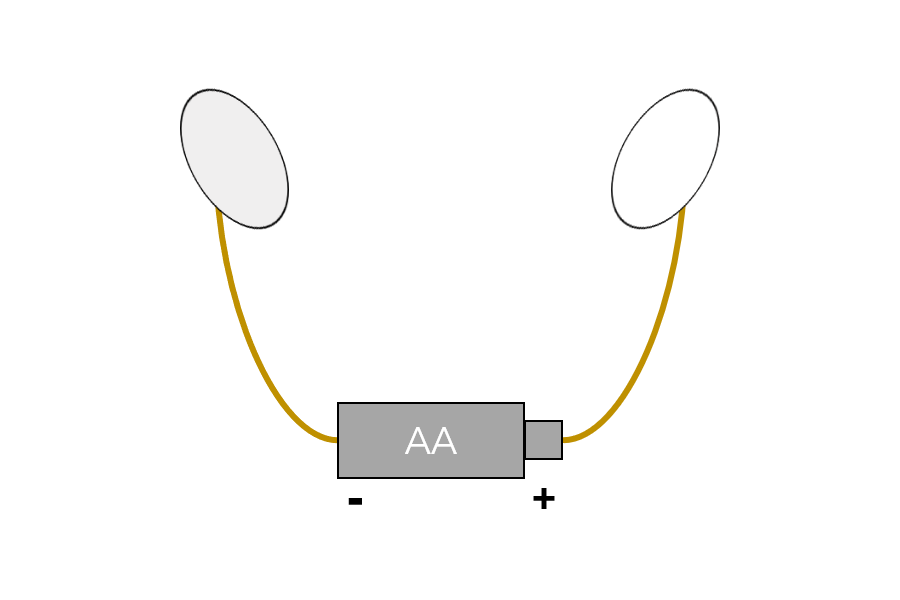What will happen?

If the two metal plates were touching each other, you would have a complete circuit with a current flowing through it.

However, because the two plates are not touching, something else is happening: the negatively charged electrons from the right plate move toward the positive terminal of the battery and are repelled from the negative terminal of the battery to the left plate.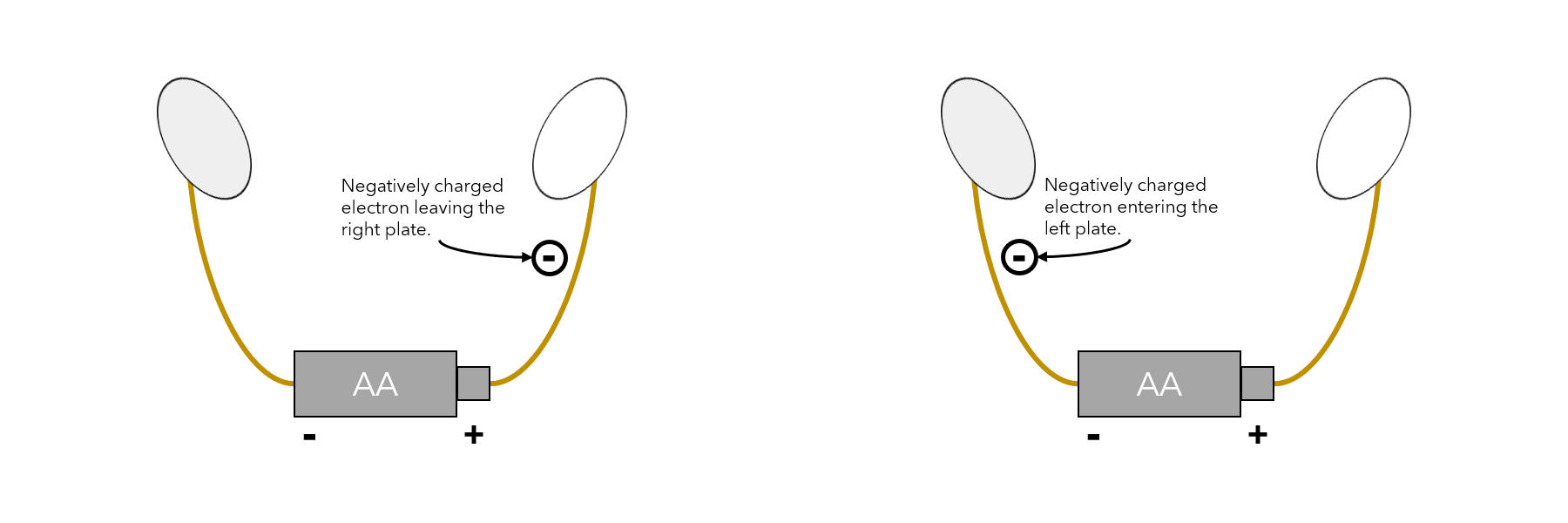As negatively charged electrons move from the right plate to the left plate, the right plate becomes positively charged (because it has fewer negatively charged electrons than positively charged protons) while the left plate becomes negatively charged (because it has more negatively charged electrons than positively charged protons).

This process will continue until the positive “pull” from the right plate matches the positive “pull” of the battery terminal.

Now, if you were to remove the battery, something strange would happen: the right plate will hold its positive charge while the left plate will hold its negative charge.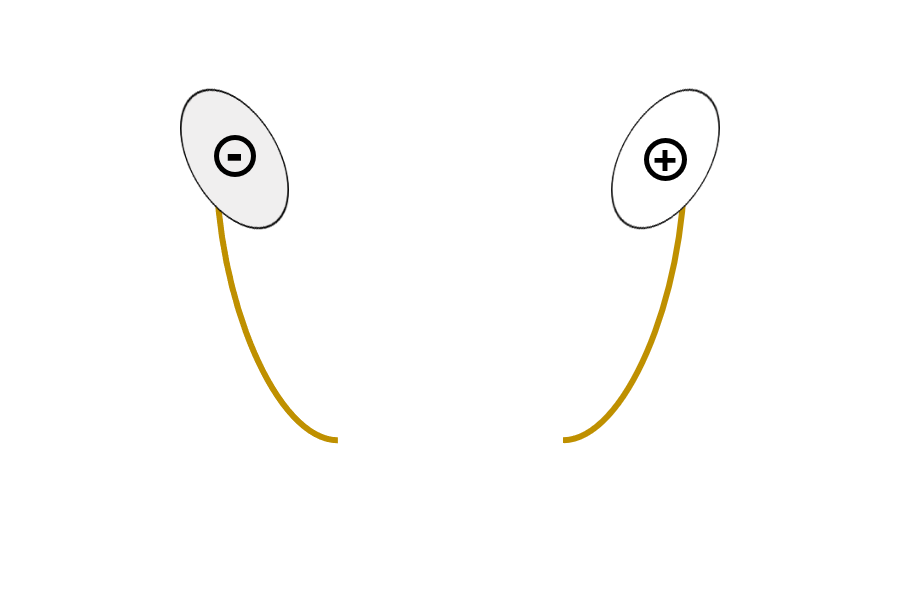This stored charge is measured in coulombs (“Q”), which represent the amount of electric charge that can be transported via a current of one ampere per second.

Because the two plates are polarized, an they create an electric field between them which holds electric potential—voltage (“V”).

Given this information, we can define capacitance (“C”) using this formula: C=Q/V.

Capacitance is measured using Farads (“f”).

In this example, the only way to change the capacitance is to alter the geometric dimensions of the plates (e.g., make them bigger) or to spread them further apart.

## What is a capacitor?

A capacitor is any component that stores electric potential in an electric field.

In our example in the previous section, the two plates connected to a battery via copper wire form a capacitor.

As capacitors store electric potential, they are commonly used to normalize the current in a circuit.

For example…

If your energy-driven medical device suffers from a voltage surge, it could be fatal to the patient—too much power to the tip of your surgical pen could cause you to cut too deep or wide.

With a capacitor, the surge could be stored in the electric field and then slowly released to ensure that just the right amount of power is being sent to the tip.

## What is interconnect capacitance?

Within the medical device industry, the term “interconnect capacitance” can be used to describe the capacitance of individual interconnect components (e.g. conductors) or the capacitance of the entire interconnect solution.

Unfortunately, there is no single equation for calculating the capacitance of an interconnect solution.

As capacitance is dependent on the geometries and distance of the charge-holding elements, different equations are used based on the shape and configuration of the interconnect.

For example…

If you have a 500mm cable that is 4mm in diameter and consists of a copper conductor that is 2mm in diameter surrounded by a PTFE jacket, you could potentially calculate the capacitance using a concentric cylinder equation:

(2 * π * ε * L) / ln(R2 / R1)

In this equation:

• “π” is the symbol for pi
• “ε” is the permittivity of PTFE (estimated at 2)
• “L” is the symbol for length (500mm)
• R2 is the radius of the cable (2mm)
• R1 is the radius of the conductor (1mm)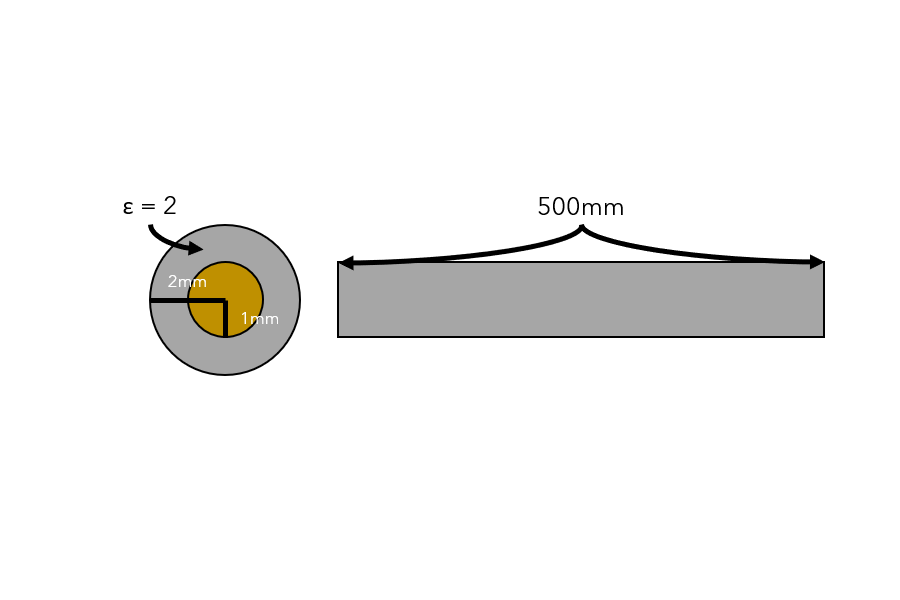So, our calculated capacitance is:

(2 * π * 2 * 500mm) / ln(2mm / 1mm) ≈ 9064.72F

If this cable was attached to a connector or another wire, we would need to use a Laplace equation to calculate the capacitance of the entire interconnect solution.

## What is fringe capacitance?

In our earlier example, when the two plates are charged, they create an electric field where electric potential is stored.

Due to the movement of electrons, this electric field doesn’t stop exactly at the edges of the plates.

Fringe capacitance” is the term commonly used to describe the extension of the electric field beyond the edge of the capacitor.Like interconnect capacitance, calculating fringe capacitance is a difficult process that utilizes formulas and functions beyond the scope of this blog post.

## What is parasitic capacitance?

Our plate example highlighted an important element of capacitance: two charged elements can create an electric field that stores electric potential.

When planned for and used correctly, capacitance can be helpful—like capacitors regulating the current of your electrosurgical device.

Unexpected capacitance, however, can be detrimental.

Unfortunately, sometimes unwanted capacitance can show up between elements because they both hold a charge.

Parasitic capacitance” is a term that is commonly used to describe this unwanted capacitance.

To better understand parasitic capacitance, consider a cable with two conductors.

If the voltage running through one of the conductors varies from the other, the imbalance will cause an electric field to develop and charge the conductors—creating unwanted capacitance that could interfere with the current flowing through the conductors.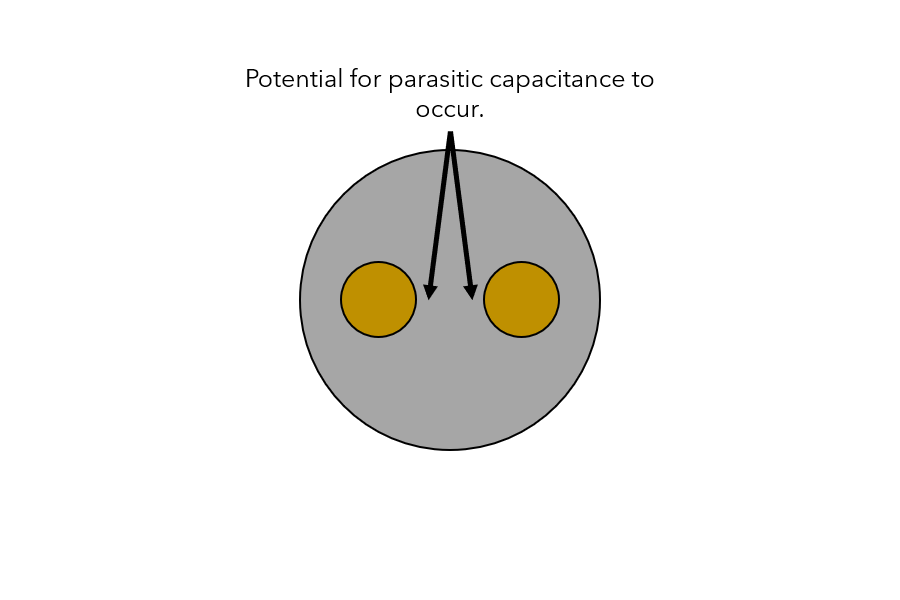Parasitic capacitance can be extremely harmful to your medical device.

Though the calculation for parasitic is complex and dependent on the configuration and material of your interconnect solutions, there are software programs and applications that can help you calculate and identify parasitic capacitance.

## What is a low capacitance interconnect cable?

As the name implies, a “low capacitance interconnect cablelow capacitance interconnect cable” is one that seeks to minimizes the interference of capacitance.

Within the medical device industry, low capacitance interconnect cables are typically associated with coaxial cable used for imaging.

Low capacitance cables have become popular because they can help improve the speed and quality of data-transmission—especially over longer distances.

Electric fields can interrupt the signal carried through a conductor.

As capacitance is stored in an electric field, cables designed with “high” capacitance can suffer from signal loss or disruption.

When it comes to medical devices, signal loss or disruption can have an adverse impact on patient outcomes.

For a cable to have “low” capacitance, it must be designed to reduce capacitance throughout the length of the wire—it requires much more than simply changing the jacketing or conductor material (remember, capacitance is a function of many things, including the distance between and geometries of the conductors).

If you’re interested in learning more about what it takes to design the right cable for your application, download our free ebook, Cable Design.